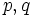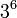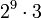# GAP:IdGroup

INTERNAL AD: Want to find out the GAP ID of a group using this wiki? If you go to the wiki page for the group, scroll down to the section titled GAP implementation, where you can find both the GAP ID and other ways to construct the group using GAP. For instance, the section dihedral group:D8#GAP implementation has information on the GAP implementation for dihedral group:D8.
You can also find summary information on all groups of a given order a by typing "groups of order a" (without quotes) into the search box.

## Definition

### Function type

IdGroup (synonym IdSmallGroup) is a GAP function with one argument, which is a group, and which outputs an ordered pair of natural numbers.

### Behavior

• If the order is finite, and GAP's library has the list of groups of that order, then GAP returns an ordered pair: the order of the group, followed by the position in which that group occurs in the list of groups with that order.
• If the group is finite but GAP does not have a list of groups of that order, GAP gives an error to that effect: it says that the group identification for groups of that size is not available.
• Similarly, if the group is infinite, GAP gives an error to the effect that the group identification for groups os size infinity is not available.

### Typical usage

IdGroup(group);

where group is a specified group.

## Available groups

IdGroup is available only for those groups that are in the SmallGroups library. However, there are some groups that are in the SmallGroups library for which the IdGroup functionality is not available, because these groups are very difficult to identify. The table below gives the list of orders for which IdGroup works. In cases where IdGroup does not work, an error message that includes the order of the group is displayed.$2^n, 1 \le n \le 8$$2,4,8,16,32,64,12,8,256$ The SmallGroup function is also available for groups of order$2^9$, but group identification is not available for this order.$3^n, 1 \le n \le 6$$3,9,27,81,243,729$ In the latest version here (not yet released as part of GAP) groups of order$3^7$ have a SmallGroup implementation but no group identification.$5^n, 1 \le n \le 5$$5,25,125,625,3125$ Groups of order$5^6$, and, in the latest version here (not yet released as part of GAP) groups of order$5^7$ have a SmallGroup implementation but no group identification.$7^n, 1 \le n \le 4$$7,49,343,2401$ Groups of order$7^5$ and$7^6$, and, in the latest version here (not yet released as part of GAP) groups of order$5^7$ have a SmallGroup implementation but no group identification.$11^n, 1 \le n \le 4$$11,121,1331,14641$ Groups of order$11^5$ and$11^6$, and, in the latest version here (not yet released as part of GAP) groups of order$11^7$ have a SmallGroup implementation but no group identification.$p^n, 1 \le n \le 4$,$p$ prime$p,p^2,p^3,p^4$ Groups of order$p^6$ have a SmallGroup implementation but no group identification.$pq^n$,$p,q$ distinct primes, and$q^n$ divides$2^8$,$3^6$,$5^5$, or$7^4$ (infinite list)
squarefree order (infinite list)
order factorizes into at most three primes (infinite list) primes are counted with multiplicity

Here are the smallest orders for which IdGroup cannot be implemented.

Order Factorization Can SmallGroup be implemented?
512$\! 2^9$ Yes
1024$\! 2^{10}$ No
1536$\! 2^9 \cdot 3$ Yes
2016$2^5 \cdot 2^3 \cdot 7$ No
2024$2^3 \cdot 11 \cdot 23$ No
2025$3^4 \cdot 5^2$ No
2040$2^3 \cdot 3 \cdot 5 \cdot 17$ No

## Related functions

### Other functions for identifying group structure

• GAP:StructureDescription: The flip side is that this does not identify a group uniquely. The plus side is that it gives a more intuitive description of the group and works for some groups outside GAP's SmallGroup library if GAP can identify the group structure.

## Examples of usage

### Single command examples

gap> IdGroup(CyclicGroup(6));
[ 6, 2 ]
gap> IdGroup(SL(2,5));
[ 120, 5 ]
gap> IdGroup(CyclicGroup(120));
4
gap> IdGroup(SmallGroup(24,7));
[ 24, 7 ]

Below is an explanation:

Command GAP output/print Other GAP functions used Explanation
IdGroup(CyclicGroup(6)); [ 6, 2 ] CyclicGroup GAP is asked to give the group ID of the cyclic group of order six, which it does: the cyclic group of order six is the second among the list of groups of order six.
IdGroup(SL(2,5)); [ 120, 5 ] SL GAP is asked for the group ID of the special linear group of degree two over field:F5.
IdGroup(CyclicGroup(120)); 4 CyclicGroup GAP is asked for the second part of the group ID of the cyclic group of order 120. It returns 4. This means that the cyclic group of order 120 has GAP ID (120, 4).
IdGroup(SmallGroup(24,7)); [ 24, 7 ] SmallGroup GAP is asked for the group ID of the group with group ID (24,7). It returns the same value back.

### Error message examples

gap> IdGroup(H);
[ 576, 8653 ]
gap> IdGroup(CyclicGroup(1024));
Error, the group identification for groups of size
1024 is not available called from
<function>( <arguments> ) called from read-eval-loop
you can 'quit;' to quit to outer loop, or
you can 'return;' to continue

Below is an explanation:

Command Error message Other GAP functions used Explanation
IdGroup(CyclicGroup(1024)); Error, the group identification for groups of size ... CyclicGroup GAP returns an error indicating that its SmallGroup library does not have groups of this order.

### Typical command sequences using this function

gap> G := SymmetricGroup(4);
Sym( [ 1 .. 4 ] )
gap> H := DirectProduct(G,G);
Group([ (1,2,3,4), (1,2), (5,6,7,8), (5,6) ])
gap> IdGroup(H);
[ 576, 8653 ]

Below is an explanation:

Command GAP output/print Other GAP functions used Explanation
G := SymmetricGroup(4); Sym( [ 1 .. 4 ] ) SymmetricGroup This defines symmetric group:S4
H := DirectProduct(G,G); Group([ (1,2,3,4), (1,2), (5,6,7,8), (5,6) ]) DirectProduct This defines a new group$H$ as$G \times G$.
IdGroup(H); [ 576, 8653 ] GAP is asked for the group ID of$H$ and returns$(576,8653)$. This indicates that the group$H$ has order$576$ and is the$8653^{th}$ group in GAP's SmallGroup library.

IdGroup can be combined with other functions, to apply it to lists of subgroups. For instance:

gap> G := SmallGroup(81,7);
<pc group of size 81 with 4 generators>
gap> List(NormalSubgroups(G),IdGroup);
[ [ 1, 1 ], [ 3, 1 ], [ 9, 2 ], [ 27, 4 ], [ 27, 4 ], [ 27, 3 ], [ 27, 5 ],
[ 81, 7 ] ]

Below is an explanation:

Command GAP output/print Other GAP functions used Explanation
G := SmallGroup(81,7); <pc group of size 81 with 4 generators> SmallGroup Constructs the group with GAP ID$(81,7)$ (this is actually wreath product of Z3 and Z3) and stores it as$G$.
List(NormalSubgroups(G),IdGroup); [ [ 1, 1 ], [ 3, 1 ], [ 9, 2 ], [ 27, 4 ], [ 27, 4 ], [ 27, 3 ], [ 27, 5 ], [ 81, 7 ] ] List, NormalSubgroups Returns the group IDs of all normal subgroups of$G$.